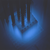0

Caglar Arslan 2 years ago updated by Pavel Holoborodko 2 years ago

is there a speed-up when we do the vector-matrix multiplication addition by using your toolbox?+1

Your question is not clear. "Speed-up" compared to what?

If we would compare it to VPA then we see ~53 times speed-up:

```>> A = vpa(rand(1000));>> x = vpa(rand(1000,1));>> tic; y = A*x; toc;Elapsed time is 1.143934 seconds.
>> A = rand(1000,'mp');>> x = rand(1000,1,'mp');>> tic; y = A*x; toc;Elapsed time is 0.021398 seconds.```+1

Your question is not clear. "Speed-up" compared to what?

If we would compare it to VPA then we see ~53 times speed-up:

```>> A = vpa(rand(1000));>> x = vpa(rand(1000,1));>> tic; y = A*x; toc;Elapsed time is 1.143934 seconds.
>> A = rand(1000,'mp');>> x = rand(1000,1,'mp');>> tic; y = A*x; toc;Elapsed time is 0.021398 seconds.```From my point of view is very important to compare multi-precision arrays creation, too!!!

```>> tic; A = vpa(rand(1000));tocElapsed time is 16.637381 seconds.

>> tic; A = rand(1000,'mp');toc
Elapsed time is 0.026866 seconds.```

So in this case, you can see ~ 619x speed up!!!

I think, the MCT is really excellent tool ... :)